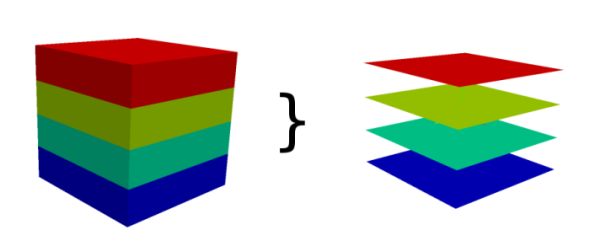## NAME

r3.to.rast - Converts 3D raster maps to 2D raster maps

## KEYWORDS

raster3d, conversion, raster, voxel

## SYNOPSIS

r3.to.rast
r3.to.rast --help
r3.to.rast [-mr] input=string output=string [type=string] [multiply=float] [add=float] [--overwrite] [--help] [--verbose] [--quiet] [--ui]

### Flags:

-m
Use 3D raster mask (if exists) with input map
-r
Use the same resolution as the input 3D raster map for the 2D output maps, independent of the current region settings
--overwrite
Allow output files to overwrite existing files
--help
Print usage summary
--verbose
Verbose module output
--quiet
Quiet module output
--ui
Force launching GUI dialog

### Parameters:

input=string [required]
3D raster map(s) to be converted to 2D raster slices
output=string [required]
Basename for resultant raster slice maps
type=string
Type of raster map to be created
Storage type for resultant raster map
Options: CELL, FCELL, DCELL
CELL: Integer
FCELL: Single precision floating point
DCELL: Double precision floating point
multiply=float
Value to multiply the raster values with
Coefficient a in the equation y = ax + b
Value to add to the raster values
Coefficient b in the equation y = ax + b

## DESCRIPTION

Converts one 3D raster map into several 2D raster maps (depends on depths). If the 2D and 3D region settings are different, the 3D resolution will be adjusted to the 2D resolution (the depths are not touched). The user can force r3.to.rast to use the 2D resolution of the input 3D raster map for the output maps, independently from the current region settings.How r3.to.rast works

### Map type conversions

Type of resulting 2D raster maps is determined by the type of the input 3D raster, i.e. 3D raster of type DCELL (double) will result in DCELL 2D rasters. A specific type for 2D rasters can be requested using the type option.

The type option is especially advantageous when the 3D raster map stores categories (which need to be stored as floating point numbers) and the 2D raster map should be also categorical, i.e. use integers. The type is set to CELL in this case.

### Modifying the values

The values in the 3D raster map can be modified prior to storing in the 2D raster map. The values can be scaled using the option multiply and a constant value can be added using the option add. The new value is computed using the following equation:
```y = ax + b
```
where x is the original value, a is the value of multiply option, b is the value of add option, and y is the new value. When multiply is not provided, the value of a is 1. When add is not provided, the value of b is 0.

## NOTES

Every slice of the 3D raster map is copied to one 2D raster map. The maps are named like output_slicenumber. Slices are counted from bottom to the top, so the bottom slice has number 1.

The number of slices is equal to the number of depths.

To round floating point values to integers when using type=CELL, the add option should be set to 0.5.